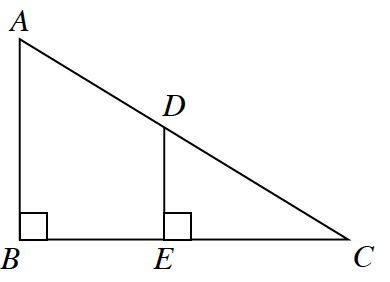### Home > CCG > Chapter 4 > Lesson 4.2.2 > Problem4-70

4-70.Are the triangles at right similar? If so, write a flowchart that justifies your conclusion. If not, explain how you know.

Draw triangles $ABC$ and $DEC$ separately. What do you notice about angles $B$ and $E$? What do you notice about angle $C$?